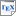[Fen13] Lu Feng. On Learning Assumptions for Compositional Verification of Probabilistic Systems. Ph.D. thesis, University of Oxford. October 2013. [pdf] [bib] [Develops several assume-guarantee techniques for compositional probabilistic verification using automatic generation of assumptions. Implements the techniques using various extensions of PRISM.] Downloads:pdf (1.98 MB)bib Links: [Google] [Google Scholar] [CiteSeer] Abstract. Probabilistic model checking is a powerful formal verification method that can ensure the correctness of real-life systems that exhibit stochastic behaviour. The work presented in this thesis aims to solve the scalability challenge of probabilistic model checking, by developing, for the first time, fully-automated compositional verification techniques for probabilistic systems. The contributions are novel approaches for automatically learning probabilistic assumptions for three different compositional verification frameworks. The first framework considers systems that are modelled as Segala probabilistic automata, with assumptions captured by probabilistic safety properties. A fully-automated approach is developed to learn assumptions for various assume-guarantee rules, including an asymmetric rule Asym for two-component systems, an asymmetric rule Asym-N for n-component systems, and a circular rule Circ. This approach uses the L* and NL* algorithms for automata learning. The second framework considers systems where the components are modelled as probabilistic I/O systems (PIOSs), with assumptions represented by Rabin probabilistic automata (RPAs). A new (complete) assume-guarantee rule Asym-Pios is proposed for this framework. In order to develop a fully-automated approach for learning assumptions and performing compositional verification based on the rule Asym-Pios, a (semi-)algorithm to check language inclusion of RPAs and an L*-style learning method for RPAs are also proposed. The third framework considers the compositional verification of discrete-time Markov chains (DTMCs) encoded in Boolean formulae, with assumptions represented as Interval DTMCs (IDTMCs). A new parallel operator for composing an IDTMC and a DTMC is defined, and a new (complete) assume-guarantee rule Asym-Idtmc that uses this operator is proposed. A fully-automated approach is formulated to learn assumptions for rule Asym-Idtmc, using the CDNF learning algorithm and a new symbolic reachability analysis algorithm for IDTMCs. All approaches proposed in this thesis have been implemented as prototype tools and applied to a range of benchmark case studies. Experimental results show that these approaches are helpful for automating the compositional verification of probabilistic systems through learning small assumptions, but may suffer from high computational complexity or even undecidability. The techniques developed in this thesis can assist in developing scalable verification frameworks for probabilistic models.